# Topic 2.3: Work, Energy, Power Flashcards Preview

## IB SL Physics > Topic 2.3: Work, Energy, Power > Flashcards

Flashcards in Topic 2.3: Work, Energy, Power Deck (57):
1

2

3

4

5

## The graph below shows the variation with displacement d of the force F applied by a spring on a cart. The work done by the force in moving the cart through a distance of 2 cm is A. 10 x 10-2 J B. 7 x 10-2J C. 5 x 10-2J D. 2.5 x 10-2 J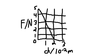6

7

## The diagram below shows the variation with displacement x of the force F acting on an object in the direction of the displacement.Which area represents the work done by the force when the displacement changes from to x1 to x2? A. QRS B. WPRT C. WPQV D. VQRT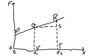8

9

10

11

## The variation with time of the vertical speed of a ball falling in air is shown below.During the time from 0 to T, the ball gains kinetic energy and loses ∆Ep gravitational potential energy. Which of the following statements is true?  A. ∆Ep is equal to the gain in kinetic energy.  B. ∆Ep is greater than the gain in kinetic energy.  C. ∆Ep is equal to the work done against air resistance.  D. ∆Ep is less than the work done against air resistance.12

## The diagram below represents energy transfers in an engine.The efficiency of the engine is given by the expressionA. Ew/EinB. Ew/EoutC. Eout/EinD. Eout/Ew13

14

## The graph below shows the variation with displacement d of the force F acting on a particleThe area that represents the work done by the force between d = 0 and d = dmax isA. s − r. B. r.C. s. D. s + r.15

## A spring is compressed by a force F.For a compression e, the force F is given by F = ke. When the compression force is removed, the spring returns to its original length in time t. The best estimate for the power developed by the spring  during its expansion isA. ke/2tB. ke/tC. ke2/2tD. ke2/t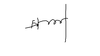16

## A     force    stretches    a    wire    that    is    fixed    at    one    end.   The    value    of    this     force    increases     from    zero    to    a     maximum value and then returns to zero. The graph below shows the variation with force F of the extension x of the wire.Which area, or areas, represents the net work done on the wire by the force?  A. Area P  B. Area Q  C. Area R  D. Area Q and area R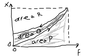17

18

19

20

## The graph below shows the variation with load F of the length L of a spring.Which of the following expressions gives the force per unit extension (the spring constant) of  the spring?A. F1/L1B. F2/L2C. (F2-F1)/L2D. (F2-F1)/(L2-L1)21

## The graph below shows the variation with displacement x of the force F acting on an object. The force F always acts in the same direction as the displacement.At point Q, the displacement is xQ and the force is FQ. Which of the following gives the work done by the force on the body as the displacement increases from zero to xQ and then returns to zero?A. Zero  B. 1/2FQxQ  C. FQxQ  D. 2FQxQ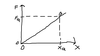22

## Water flows out from a tank down a pipe, as shown belowThe pipe is always full of water. Which of the following gives the change in the kinetic energy and in the gravitational potential energy  of the water as the water flows down the pipe?                     kinetic energy                  gravitational potential energyA.                  constant                           decreasesB.                  constant                           increasesC.                  increases                         decreasesD.                  increases                         increases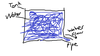23

24

25

26

## A weight is suspended from a spring. The variation with weight of the length of the spring is shown below.What is the value of the spring constant (force constant) of the spring?  A. 0.4 N cm-1  B. 0.5 N cm-1  C. 2.0 N cm-1  D. 2.5 N cm−127

## The point of action of a constant force F is displaced a distance d. The angle between the force and the direction of the displacement is θ , as shown below.Which one of the following is the correct expression for the work done by the force?  A. Fd sinθ  B. Fd sinθ  C. Fd cosθ  D. Fd tanθ28

29

30

31

32

33

34

35

36

37

38

39

40

41

42

43

44

45

46

47

48

49

## A ball falls vertically and bounces off the ground. Immediately before impact with the ground the speed of the ball is u. Immediately after leaving the ground the speed is v. Which of the following expressions is the ratio of kinetic energy lost on collision/kinetic energy immediately before collision ?A.     v/u B. 1- (v/u) C.     (v/u)2D.     1- (v/u)250

## A railway engine of mass m moves along a horizontal track with uniform speed v. The total resistive force acting on the engine is F.Which of the following is the power of the engine?A.    F/mv B.     Fv C.  mv/F D.  v/F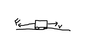51

## A ball is thrown vertically upwards and comes down again. Air resistance is negligible. Which of the following graphs shows how the gravitational potential energy EP varies with time t ?52

53

54

55

56

57

## A constant force acts on a mass that is initially at rest. Which of the following graphs best shows how the kinetic energy EK of the mass changes with the work W done on the mass? Friction is negligible.